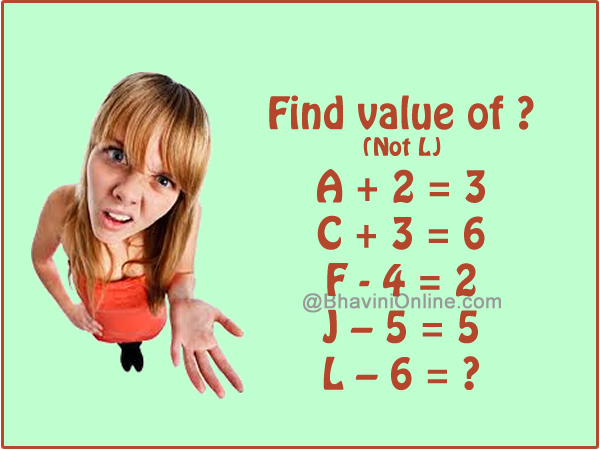# Challenge Your Brain: If A + 2 = 3 Then L – 6 = ?

Challenge your brain with this sequence riddle.

See the equations and try to find the connection between them.

Once you have done that it will be easy to find the value of ?

A + 2 = 3

C + 3 = 6

F – 4 = 2

J – 5 = 5

L – 6 = ?Leave your answers in the comment section and if you want to have fun with this, share it with your friends on Facebook and WhatsApp.

The values of the letters are actually the corresponding number it has in that order. If you number A = 1, B = 2 so on till Z = 26.

 A B C D E F G H I J K L M N O P Q R S T U V W X Y Z 1 2 3 4 5 6 7 8 9 10 11 12 13 14 15 16 17 18 19 20 21 22 23 24 25 26

So if we substitute these values in above equations;

A + 2 = 1 + 2 = 3

C + 3 = 3 + 3 = 6

F – 4 = 6 – 4 = 2

J – 5 = 10 – 5 = 5

Therefore;

L – 6 = 12 – 6 = 6